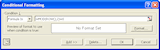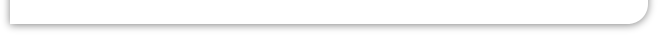Automatically updating a chart in MS Excel? When using Excel to display data from a datalogger or device in real- time, you may find it useful to also automatically update a chart in Excel. Suppose you wanted to plot data in column A against the data in column B. You could simply insert a chart with the range =\$A:\$B As the data arrives from Windmill DDE Panel, the chart will automatically update. However, if the rows of data exceed the original size of the worksheet, the chart will stop. You can get round this by using Excel's CountA and Offset functions. CountA gives you the number of non- blank cells in a column. With Windmill data this equates to the number of rows of data (and headings). Offset returns a cell reference according to your settings. Using CountA as the height of the column gives a reference to the bottom row. This reference will automatically update when new data arrives. For example, this is the syntax of Offset: OFFSET(reference, rows, cols, height, width) which becomes: OFFSET(Sheet1!\$A\$1,0,0,COUNTA(Sheet1!\$A:\$A)) (assuming your data is in a worksheet called "Sheet1"). To implement this, using the previous example of plotting column A against column B. 1. Create 2 named ranges called ColA and ColB (for example). For Column A, from the Insert menu choose Name then Define. In the names box type ColA and in the Refers to box type =OFFSET(Sheet1!\$A\$1,0,0,COUNTA(Sheet1!\$A:\$A)) Press Add For Column B, in the names box type ColB and in the Refers to box type =OFFSET(Sheet1!\$B\$1,0,0,COUNTA(Sheet1!\$B:\$B)) Press Add and close the dialogue box.Conditional Formatting Box 2. Insert a chart plotting Column A against Column B. 3. We now need to edit the chart so it automatically updates. Select the chart and double-click the plotted data series. Replace the current X Values with =Sheet1!ColA In the Name and Values options, delete the current Name and replace the Y values with =Sheet1!ColB (In the in the formula bar this will look like: =SERIES(,Sheet1!ColA,Sheet1!ColB,1) 3. Click off the chart and start importing data into Excel. The chart will continually update no matter how many rows of data you collect. The formula in step 4: =MOD(ROW(),2)=0, checks whether the row number divided by 2 has a remainder of 0. If this is true, the row is coloured in. If false the row is left as it is.